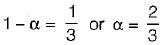Courses

# Chopper - 2

## 10 Questions MCQ Test Topicwise Question Bank for Electrical Engineering | Chopper - 2

Description
This mock test of Chopper - 2 for Electrical Engineering (EE) helps you for every Electrical Engineering (EE) entrance exam. This contains 10 Multiple Choice Questions for Electrical Engineering (EE) Chopper - 2 (mcq) to study with solutions a complete question bank. The solved questions answers in this Chopper - 2 quiz give you a good mix of easy questions and tough questions. Electrical Engineering (EE) students definitely take this Chopper - 2 exercise for a better result in the exam. You can find other Chopper - 2 extra questions, long questions & short questions for Electrical Engineering (EE) on EduRev as well by searching above.
QUESTION: 1

### A d.c. chopper circuit connected to a 100 V d.c. source supplies an inductive load having 40 mH in series with a resistance of 5 Ω A freewheeling diode is placed across the load. The load current varies between the limits of 10 A and 12 A. The time ratio (Ton/Toff) of the chopper is

Solution:

The average value of load current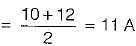The maximum value of load current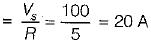Now, the average value of the voltage is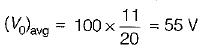Also,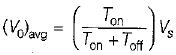or,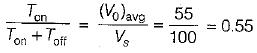or,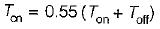or,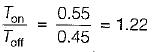QUESTION: 2

### A step-up chopper is used to deliver load voltage of 500 V from a 220 V d.c. source. If the blocking period of the thyristor is 80 μs, the required pulse width is

Solution:

Given, \/s = 220V, V0 = 500V
and Toff = 80 μs
For a step-up chopper, output voltage is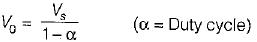or,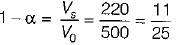or,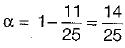or,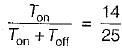or, 25 Ton = 14 Ton + 14 Toff
or, 11 Ton = 14 Toff
or,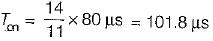QUESTION: 3

### A 220 V dc shunt motor runs at 1500 rpm at no-load. The motor is fed through a type-A chopper. If the armature resistance is 1 Ω and duty cycle of chopper is 60%, the speed of the motor when it draw a current of 20 A would be approximately equal to

Solution: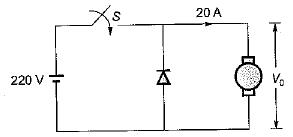The output voltage,
V0 = αVs = 0.6 x 220 - 132 V
∴ Back emf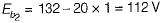At no- load, N1, = 1500 rpm and Eb1 = 220 V Now, Eb α N
∴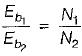or,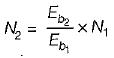or,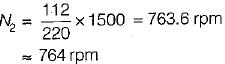QUESTION: 4

If the motor in the previous problem is driven by an active load at 1710 rpm, then the type of chopper suitable for speed control and its duty cycle for an input voltage of 250 V will be respectively

Solution:

As the motor is running at 1710 rpm its back emf is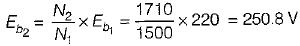Since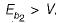therefore current flows in the reverse direction and hence second quadrant chopper will be used with the motor.
Now,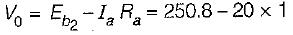= 230.8 volt
Also, V0 = (1 - α) Vs
or, 230.8 = (1 - α) x 250
or, 1 - α = 0.9232 or α = 0.0768
or, α = 7.68% = 7.7%

QUESTION: 5

A four-quadrant chopper is driving a separately excited dc motor load. The motor parameters are R = 0.1 Ω, L = 10 mH. The supply voltage is 200 V d.c. If the rated current of the motor is 10 A with Eb = 150 V and motor is driving the rated torque, then it is operating under

Solution:

For a four-quadrant chopper, the average voltage in all the four-modes is given by
V0 = 2 Vs (α - 0.5)
The average curreni,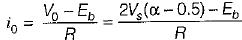or,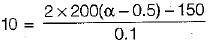or,    α = 0.877
Since α > 0.5, the motor is operating undi
forward motorina mode.

QUESTION: 6

A single-quadrant type A chopper is operating with the following specifications:
Ideal battery of 220 V;
on-time ton - 1 ms;
off-time foff = 1.5 ms
The ripple and form factors will be respectively given by

Solution:

For a type-A chopper, average output voltage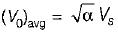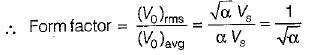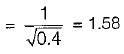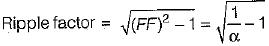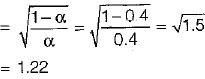QUESTION: 7

Match List-1 (Chopper circuit) with List-II (Characteristic) and select the correct answer using the codes given below the lists: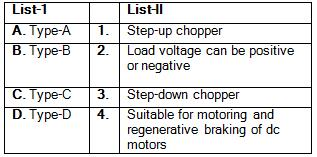Codes: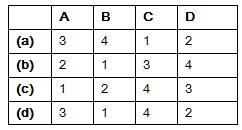Solution:

• Type-A chopper is also called “step-down chopper” as average output voltage V0 is always less than the input dc voltage Vs.
• Type-B chopper is also called “step-up chopper” as load voltage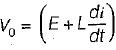which is more than source voltage Vs.
• Type-C chopper configuration is suitable for motoring and degenerative braking of dc motors.
• Type-D chopper characteristic is shown below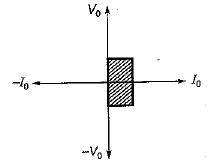QUESTION: 8

Consider the following statements:
1. DC chopper can be used in both dc and ac drives.
2. For four-quadrant operation dual converter is required.
3. Output voltage from a chopper circuit depends both on load current and duty cycle
4. In a step-down source, current can be discontinuous if duty cycle is low.
Which of the statements given above are correct?

Solution:

• DC choppers can be used in only dc drives.
• Output voltage from a chopper circuit depends only on duty cycle and is independent of load current.
Hence, statements 1 and 3 are not correct.

QUESTION: 9

For the type-A chopper shown below, the voltage drop across the chopper when it is on is 2 volt. If the duty cycle is 0.4, then the chopper efficiency would be approximately equal to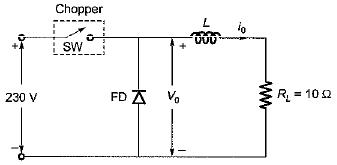Solution:

Given, Vs = 230 V
Load resistance, RL = 10Ω   α = 0.4
When chopper is ON,
output voltage = (V- 2) volt
When chopper is OFF, output voltage = 0 volt
Average output voltage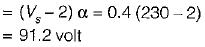Rms output voltage,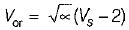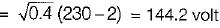Power output delivered to load is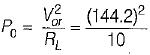= 2079.364 Watt
Power input to chopper;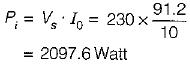Chopper efficiency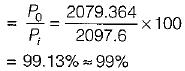QUESTION: 10

A boost-regulator has an input voltage of 5 V and the average output voltage of 15 V, The duty cycle is

Solution:

Boost-regulator is a step-up chopper.
Output voltage,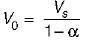or,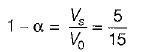or,